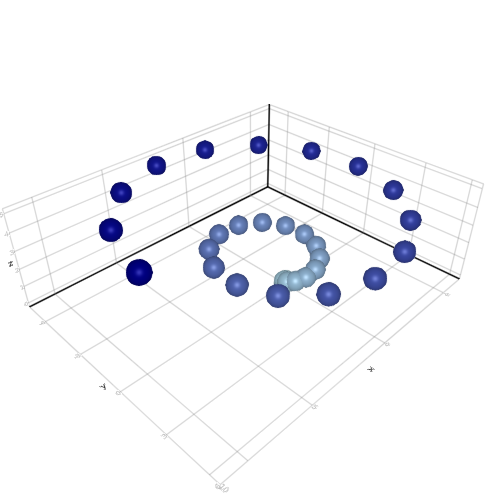## 3D

```

using AbstractPlotting

x = [2 .* (i/3) .* cos(i) for i in range(0, stop = 4pi, length = 30)]
y = [2 .* (i/3) .* sin(i) for i in range(0, stop = 4pi, length = 30)]
z = range(0, stop = 5, length = 30)
meshscatter(x, y, z, markersize = 0.5, color = to_colormap(:blues, 30))

```